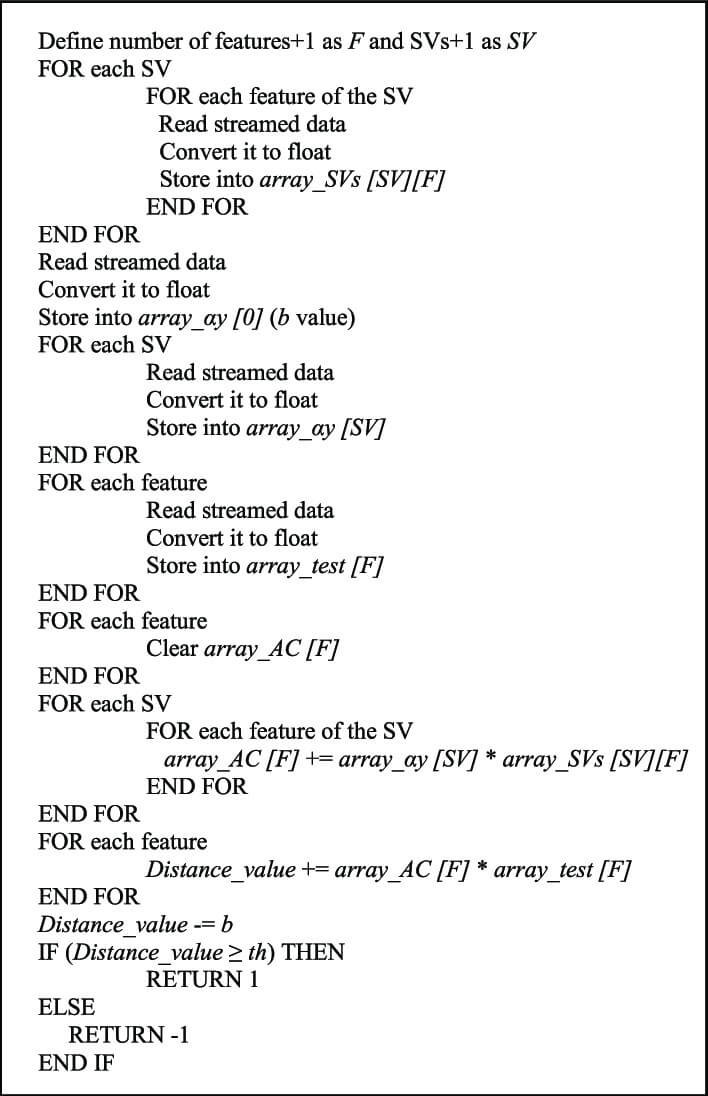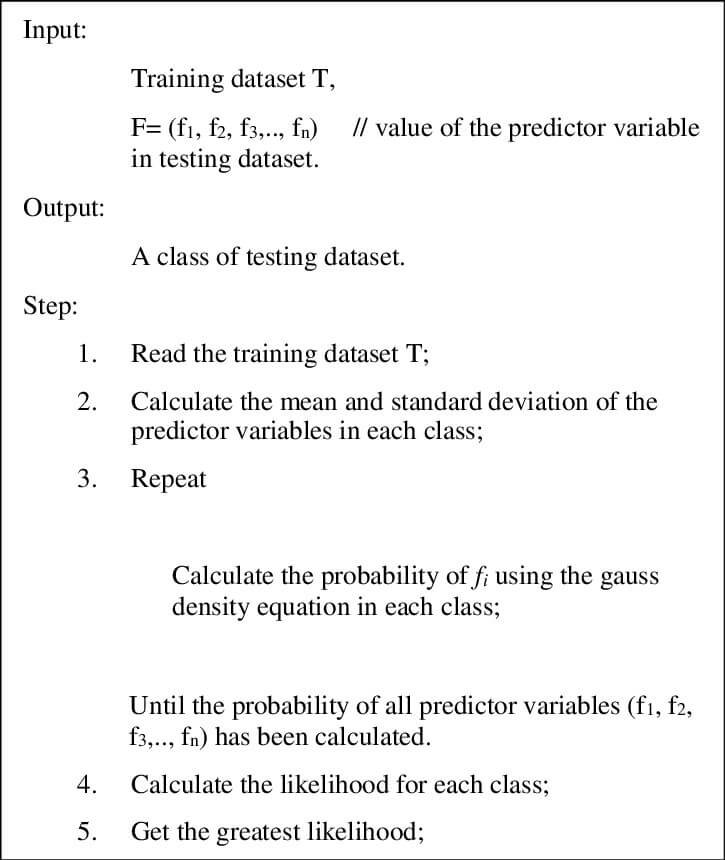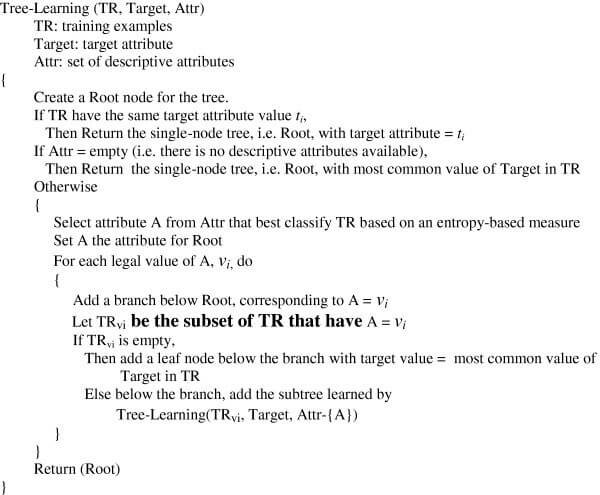# 5 powerful machine learning algorithmsMachine learning (ML) is the study of computer algorithms that improve automatically through experience. It is seen as a subset of artificial intelligence.

Machine learning algorithms build a mathematical model based on sample data, known as “training data”, in order to make predictions or decisions without being explicitly programmed to do so.

Machine learning algorithms are used in a wide variety of applications, such as email filtering and computer vision, where it is difficult or infeasible to develop conventional algorithms to perform the needed tasks.

Machine learning is closely related to computational statistics, which focuses on making predictions using computers. This is also the best environment setup for machine learning projects.

In this article, we are going to list the top 5 most used algorithms in Machine Learning that are used in many projects and give good results.

### 1. Support Vector Machine (SVM)

In machine learning, support vector machines (SVMs) are supervised learning models with associated learning algorithms that analyze data used for classification and regression analysis.

The Support Vector Machine (SVM) algorithm is a popular machine learning tool that offers solutions for both classification and regression problems.

In addition to performing linear classification, SVMs can efficiently perform a non-linear classification using what is called the kernel trick, implicitly mapping their inputs into high-dimensional feature spaces.Image 1: SVM pseudocode

### 2. K-Means

K-means clustering is a method of vector quantization, originally from signal processing, that aims to partition n observations into k clusters in which each observation belongs to the cluster with the nearest mean (cluster centers or cluster centroid), serving as a prototype of the cluster.

The algorithm has a loose relationship with the k-nearest neighbor classifier, a popular machine learning technique for classification that is often confused with k-means due to the name.

Applying the 1-nearest neighbor classifier to the cluster centers obtained by k-means classifies new data into the existing clusters.Image 2: K-Means pseudocode

### 3. Naïve Bayes

In statistics, Naïve Bayes classifiers are a family of simple “probabilistic classifiers” based on applying Bayes’ theorem with strong (naïve) independence assumptions between the features. They are among the simplest Bayesian network models. But they could be coupled with Kernel density estimation and achieve higher accuracy levels.Image 3: Naïve Bayes pseudocode.

### 4. Decision Tree

A decision tree is a decision support tool that uses a tree-like model of decisions and their possible consequences, including chance event outcomes, resource costs, and utility. It is one way to display an algorithm that only contains conditional control statements.

Decision trees are commonly used in operations research, specifically in decision analysis, to help identify a strategy most likely to reach a goal, but are also a popular tool in machine learning.Image 4: Decision Tree pseudocode

### 5. K-nearest neighbors (KNN)

In pattern recognition, the k-nearest neighbor algorithm (k-NN) is a non-parametric method proposed by Thomas Cover used for classification and regression. In both cases, the input consists of the k closest training examples in the feature space. The output depends on whether k-NN is used for classification or regression:

In k-NN classification, the output is a class membership. An object is classified by a plurality vote of its neighbors, with the object being assigned to the class most common among its k nearest neighbors (k is a positive integer, typically small). If k = 1, then the object is simply assigned to the class of that single nearest neighbor.

In k-NN regression, the output is the property value for the object. This value is the average of the values of k nearest neighbors.

k-NN is a type of instance-based learning, or lazy learning, where the function is only approximated locally and all computation is deferred until function evaluation. Since this algorithm relies on distance for classification, normalizing the training data can improve its accuracy dramatically.

Both for classification and regression, a useful technique can be to assign weights to the contributions of the neighbors, so that the nearer neighbors contribute more to the average than the more distant ones.

For example, a common weighting scheme consists in giving each neighbor a weight of 1/d, where d is the distance to the neighbor.Image 5: KNN classification pseudocode

Conclusion

These are our top 5 Machine Learning algorithms that will solve your problems. Most of them are easy to understand and implement and yet very powerful if you are using them in the right situation and if you tune them nicely.

Obviously, there are tons of other popular algorithms, like Linear Regression, Random Forest, Gradient Boosting algorithms, and so on, but we will leave them for future articles.

If you are new to Machine Learning, Deep Learning, Computer Vision, Data Science or just Artificial Intelligence, in general, we will suggest you some of our other articles that you might find helpful, like:

Here’s another interesting article about Types of Machine Learning Algorithms from the 7wdata blog.

Like with every post we do, we encourage you to continue learning, trying and creating.

Note: the cover picture is borrowed from datasciencedojo.com.